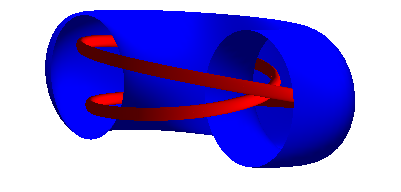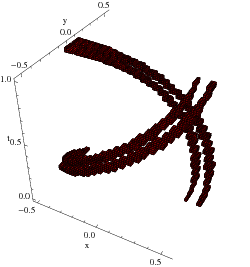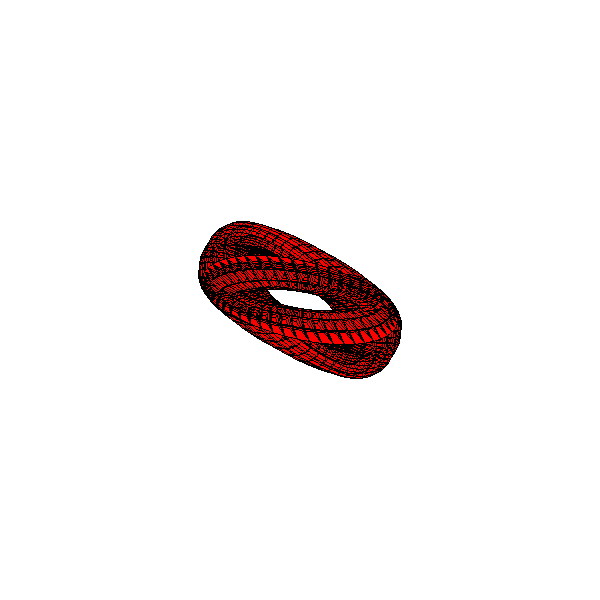The CAPD library has been used in several articles in which chaotic dynamics, bifurcations, heteroclinic/homoclinic solutions and periodic orbits were studied.

 Uniformly hyperbolic attractors for Poincare maps. Hyperbolic systems contracting volume often manifest robust attractors. There are well known model maps that admits hyperbolic attractor, like Smale map or Plykin map on the sphere. It general, it is rather difficult to prove analytically that a given map or an ODE possesses a hyperbolic chaotic invariant set. We proposed an algorithm for computer assisted verification if a map possesses uniformly hyperbolic invariant set. As a test case we applied the algorithm to the model Smale map S(x,y,t) = (0.1x+0.5cos(t),0.1y+0.5sin(t),2t) where (x,y,t)∊ D2⨯𝓢1 to prove the existence of an uniformly hyperbolic attractor. The other example we considered is the well known Henon map Ha,b(x,y) = (a - x2 + by, x) The hyperbolicity of this map for various parameter values has been studied by many authors, also by means of computer assisted approach (for example, Hruska, Arai, Mazur and Tabor). Our aim was to see if the proposed method works in this relatively easy example. The main motivation for developing new algorithms was to prove the Kuznetsov conjecture. In 2005 he proposed a non-autonomous system that apparently possesses hyperbolic attractor of the Smale-Williams type in the Poincare map. The system is given by x' = ω u u' = -ω x + (A cos (2πt/T)-x2)u + (ε/ω)y cos(ω t) y' = 2ω v v' = -2ω y + (-A cos (2πt/T)-y2)v + (ε/(2ω))x 2 Theorem. Consider the above system with the parameter values ω=2π, A=5, T=6, ε=0.5. Let P : ℝ4→ℝ4 be a Poincare map defined as a time T shift along the trajectories. There exists a compact, connected and explicitly given set B such that B is positive invariant with respect to P P is uniformly hyperbolic on the set A = ⋂n>0Pn(B) with 1 positive and three negative Lyapunov exponents the attractor A is nontrivial - it is compact, connected and contains continuum points. In particular it contains fixed point and a period two orbit for P The set B and an animation of the toroidal attracting domain is shown below. Reference: D. Wilczak, Uniformly hyperbolic attractor of the Smale-Williams type for a Poincare map in the Kuznetsov system, SIAM Journal on Applied Dynamical Systems, Vol. 9, No. 4 1263-1283 (2010).(Left) The domain X=D2⨯𝓢1 and its image S(X) under the Smale map. (Right) An enclosure of the same attractor obtained from our algorithm. Notice, the planes t=0 and t=1 should be identified.A rigorous enclosure of the hyperbolic invariant set for the Henon map with a=-1 and b=5.4.A rigorous enclosure of the hyperbolic attractor in the Kuznetsov system projected onto two pairs of variables.Animation of 4-dimensional attracting domain. The file is being loaded (ca 3.2MB) and the animation will start automatically, provided you have JavaScript enabled. Otherwise, click the picture to see the animation.